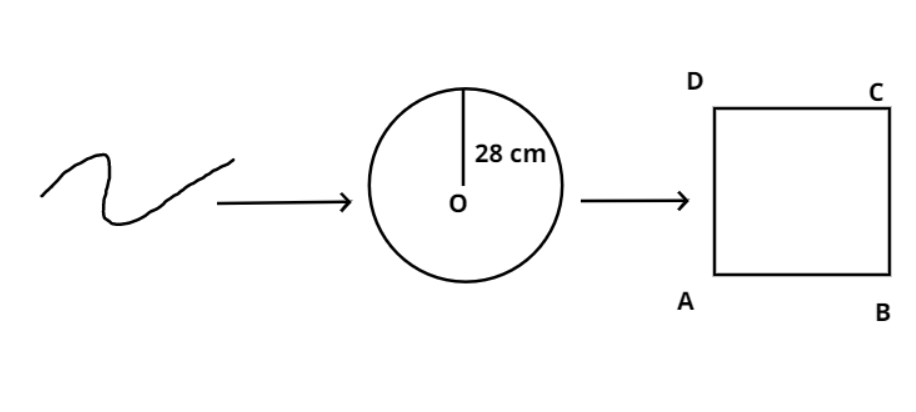Courses
Courses for Kids
Free study material
Free LIVE classes
MoreLIVE
Join Vedantu’s FREE Mastercalss

# A wire is looped in the form of a circle of radius 28 cm. If it is re-bent into a square form. Determine length of side of the square.$(a){\text{ 44 cm}} \\ (b){\text{ 45 cm}} \\ (c){\text{ 46 cm}} \\ (d){\text{ 48 cm}} \\$Verified
364.5k+ views
Hint: It is given that the wire is bent in the form of a circle of radius 28 cm, this means the entire length of the wire must be used as a circumference to this circle. Now this circle is re-bent into square form meaning that the entire circumference of the circle is not the sum of all 4 sides of the square. Use this concept to find out the length of the side of the square.Given radius of the circle, r = 28 cm………………… (1)
Circumference of a circle with radius r is given as $Circum = 2\pi r$………………. (2)
Putting the values in equation (2) from equation (1) we get,
$Circum = 2\pi (28) = 2 \times \dfrac{{22}}{7} \times 28 = 176{\text{ cm}}$…………………. (3)
Now this circumference will be equal to all 4 sides of the square as this circle is re-bent into a square so,
Length of side of square = $= \dfrac{{Circumference{\text{ of circle}}}}{{Total{\text{ side of square}}}} = \dfrac{{176}}{4} = 44cm$
Thus option (a) is the right answer.

Note: Whenever we face such type of problems the key concept is about the understanding of the problem statement, moreover the basic formula about the area, circumference etc, of various basic shapes like circle, square also help in getting the right answer.
Last updated date: 02nd Oct 2023
Total views: 364.5k
Views today: 6.64k